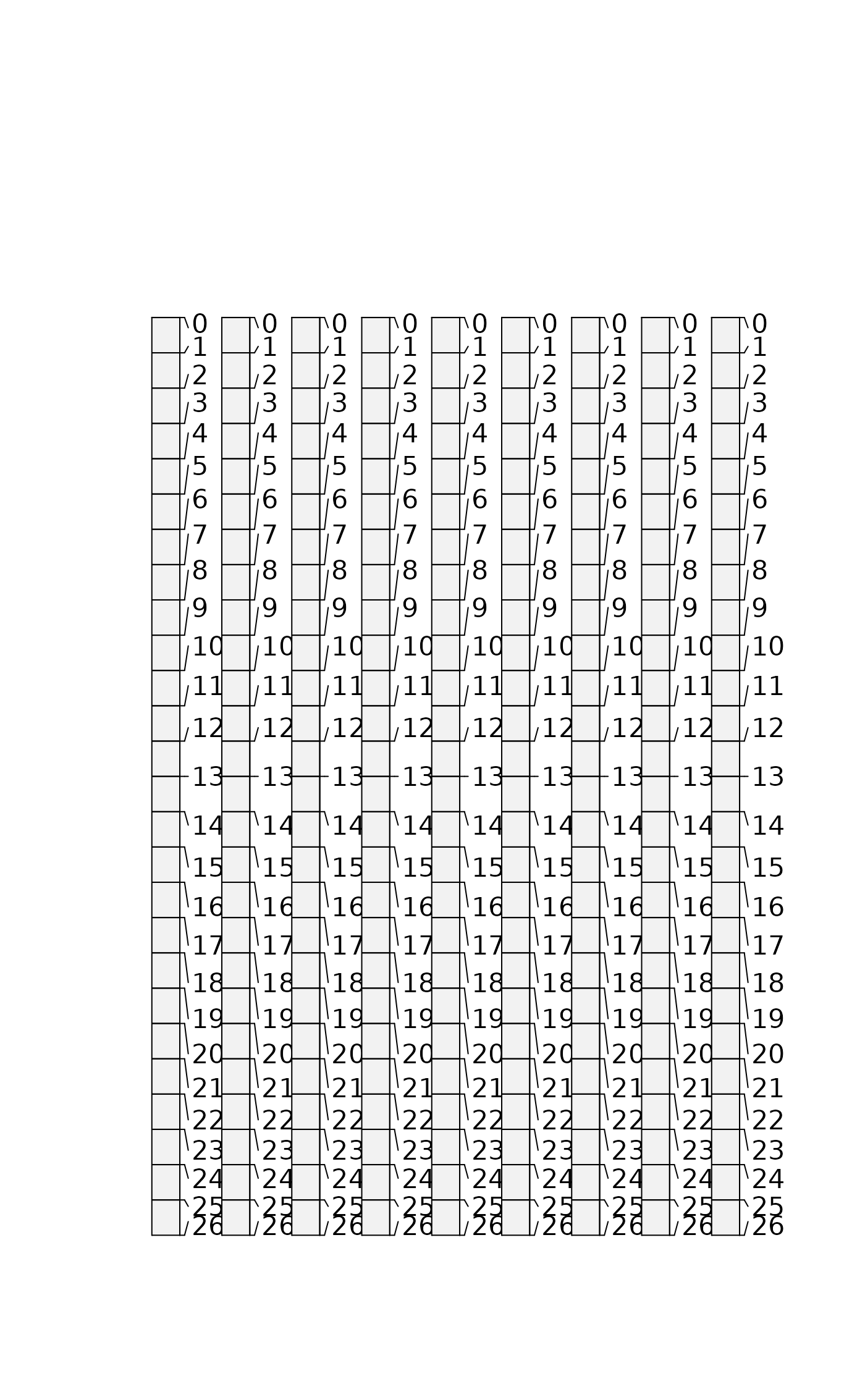Soil profile data from Pinnacles National Monument, CA.

## Format

A data frame with 60 observations on the following 21 variables.

group

a numeric vector

id

a character vector

top

a numeric vector

bottom

a numeric vector

bound_distinct

a character vector

bound_topography

a character vector

name

a character vector

texture

a character vector

prop

a numeric vector

a character vector

structure_size

a character vector

structure_type

a character vector

stickiness

a character vector

plasticity

a character vector

field_ph

a numeric vector

hue

a character vector

value

a numeric vector

chroma

a numeric vector

## Examples


data(sp1)
# convert colors from Munsell to hex-encoded RGB
sp1$soil_color <- with(sp1, munsell2rgb(hue, value, chroma)) # promote to SoilProfileCollection depths(sp1) <- id ~ top + bottom site(sp1) <- ~ group # re-sample each profile into 1 cm (thick) depth slices # for the variables 'prop', 'name', 'soil_color' # result is a SoilProfileCollection object s <- dice(sp1, 0:25 ~ prop + name + soil_color) #> dropping horizons with invalid depth logic, see metadata(x)$removed.horizons
#> filling gaps left by HzDepthLogicSubset

# plot, note slices
plot(s)# aggregate all profiles along 1 cm depth slices,
# using data from column 'prop'
s1 <- slab(sp1, fm= ~ prop)
#> Note: aqp::slice() will be deprecated in aqp version 2.0
#> --> Please consider using the more efficient aqp::dice()

# check median & IQR
library(lattice)
xyplot(top ~ p.q50 + p.q25 + p.q75,
data=s1, type='S', horizontal=TRUE, col=1, lty=c(1,2,2),
panel=panel.superpose, ylim=c(110,-5), asp=2)